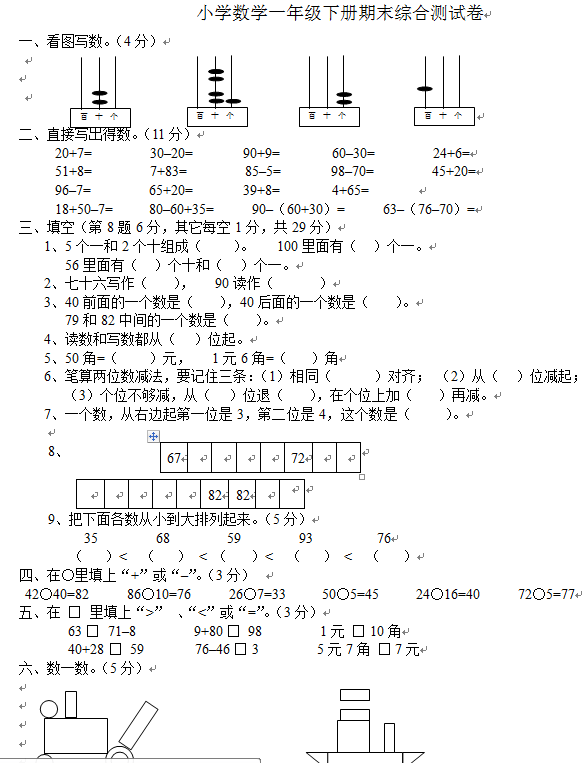ceomax主题已启用，当前站点还没有验证正版主题授权，暂不可使用 前往授权激活 获取正版授权
【DOC文档25页】小学数学一年级下册期末试卷(7套)北师大版A4电子版资料_可直接打印_会员免费下载 - 悟思学_领悟_思考_学习

【DOC文档25页】小学数学一年级下册期末试卷(7套)北师大版A4电子版资料_可直接打印_会员免费下载

324

（     ）       （     ）       （     ）       （     ）

20+7=        30–20=       90+9=       60–30=        24+6=

51+8=        7+83=        85–5=       98–70=        45+20=

96–7=        65+20=       39+8=       4+65=

【DOC文档25页】小学数学一年级下册期末试卷(7套)北师大版A4电子版资料_可直接打印_会员免费下载相关资料253 5687 2457 2458 2

• 0 +

访问总数

• 0 +

会员总数

• 0 +

文档总数

• 0 +

今日发布

• 0 +

本周发布

• 0 +

运行天数